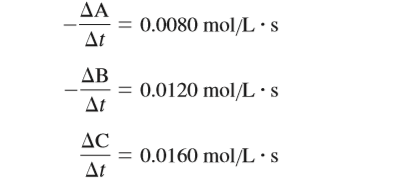# Problem: Consider the general reaction aA + bB → cC and the following average rate data over some time period Δt:Determine a set of possible coefficients to balance this general reaction.

🤓 Based on our data, we think this question is relevant for Professor Du's class at UGA.

###### FREE Expert Solution

We are asked to determine a set of possible coefficients to balance the given general reaction:

aA + bB → cC

Given:###### Problem Details

Consider the general reaction

aA + bB → cC

and the following average rate data over some time period Δt:Determine a set of possible coefficients to balance this general reaction.